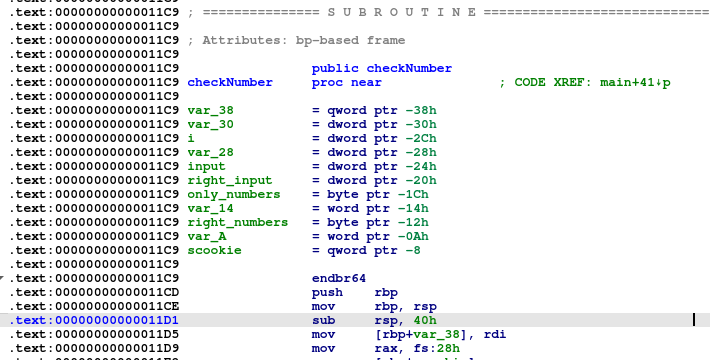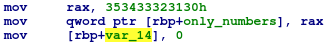# Guessing the random number using a simple stack overflow

This is a solution walkthrough to the guess challenge https://platform.intervee.io/challengeinfocard/guess

The virtual interviewer says: “Find an input, so the command ‘./guess.o [your_input]’ will print the good message.

When typing ls, you can see both the source code and the executable itself.

``````#include <stdio.h>
#include <string.h>
#include <stdlib.h>
#include <time.h>

#include <stdio.h>
#include <string.h>
#include <stdlib.h>
#include <time.h>

int checkNumber(char * number) {
int input = 0;
int right_input = 0;
int j = 0;
char only_numbers = "012345";
char right_numbers = "012345";

srand ( time(NULL) );

for (int i = 0 ; i < 9 ; i++) {
right_numbers[i] = rand()%10 + '0';
}

for (int i = 0 ; i < 9 ; i++) {
if (number[i] >= '0' && number[i] <= '9') only_numbers[++j] = number[i];
}

input = atoi(only_numbers);
right_input = atoi(right_numbers);

if (input == right_input)  return 1;
printf("Wrong - the random number was %s. You will need a little bit more luck in guessing.\n",right_numbers);

return 0;
}
int main(int argc, char * argv[]) {

int correct = 0;
if (argc != 2) {
printf("Wrong number\n");
return 0;
}
correct = checkNumber(argv);

if (correct) {
printf("Good!!\n");
}
return 0;
} ``````

Let’s focus on this piece of code (take a few minutes to find the vulnerability, and then continue reading):

``````	char only_numbers = "012345";
char right_numbers = "012345";

srand ( time(NULL) );

for (int i = 0 ; i < 9 ; i++) {
right_numbers[i] = rand()%10 + '0';
}

for (int i = 0 ; i < 9 ; i++) {
if (number[i] >= '0' && number[i] <= '9') only_numbers[++j] = number[i];
}
``````

Both only_numbers and right_number are 10 bytes in length and there are two loops that copy 9 bytes into a 10-byte length. Not a problem so far.

However, pay attention to this code:
`only_numbers[++j] = number[i];`
Since there is a “++j” and not “j++”, it means that we first increment j before assigning to only_numbers the value number[i].
That means that instead of copying to bytes 0-8, we copy to 1-9.
Only_numbers is a null-terminated string, so the value only_numbers is null. We can overwrite it with our value.

What happens if we overwrite the null byte of only_numbers?
If only_number doesn’t have a null terminator, then the function atoi(only_numbers) can’t know when/where to stop reading and will continue to read the bytes in the stack until it reaches the null byte of random_numbers.

The solution:
If number (your input) is 000000000, then atoi will return the same number both for only_number and random_number! Boom 🙂

Bonus (not necessary for solving this challenge):
Maybe you are asking yourself, is right_number located in the stack directly after only_numbers? Can you prove it? Let’s take a look:As you can see (don’t worry if not, IDA is relevant only for challenges with 50+ score), there are 3 vars one after another: only_numbers,var_14,right_numbers.
Only_numbers has a length of 8 bytes (0x1c-0x14), var_14 (2 bytes), and then right_numbers.
Var_14 should be a part of only_number. It’s a small mistake of IDA that treated those vars separately.
Probably it’s because we initialize only_numbers with “12345” which is 6 bytes long (5+null), but the compiler uses 8 bytes assisgmnet for this and added a third assessment for the last bytes of only_numbers in a separate line.That’s why IDA thought that var_14 is an entirely different variable, although there is no additional direct access to this variable except for initializing it with zero.

You can use this challenge on your candidates or even employees using the create wizard button. It’s free.
https://platform.intervee.io